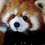# Almost-integers

You'll find something interesting if you take the golden ratio, $$\phi = \frac{1 + \sqrt{5}}{2}$$, to high powers:

$\phi^6 = 17.944$ $\phi^{10} = 122.992$ $\phi^{15} = 1364.0007$

It seems as if large exponents of $\phi$ come arbitrarily close to integer values. In fact, $\phi$ isn't the only number which does this. For example, $(2+ \sqrt{3})^{5} = 723.999$. This is no coincidence.

These numbers - known as Pisot-Vijayaraghavan numbers - produce "almost-integers" when raised to high powers. I'll give a quick sketch of why this is, as well as an easy way to find other numbers with this property:

We can take a number $q = a + \sqrt{b}$, where $a$ and $b$ are integers, and consider how it behaves under an operation called Galois conjugation. Essentially, this is just a more general version of what you may already know as complex conjugation. To find the Galois conjugate of $q$, simply change the sign of the square root term: $q* = a - \sqrt{b}$. This is equivalent to setting $q$ to be the root of the polynomial $x^2 - 2ax + a^2 - b = 0$ and finding the second root, or $q*$.

A few properties of Galois conjugation can be easily deduced, and are already implicit in the quadratic equation above:

$q + q* = 2a$ $qq* = a^2 - b$ $q^2 + q*^2 = 2a^2 + 2b$ $q^n + q*^n = \sum \binom{n}{k} a^{n-2k}b^{2k}$

The last equality is important here. Notice especially that these four equations all produce integers results on the righthand side. Now, whenever $|q*| < 1$, the second term of $q^n + q*^n$ becomes arbitrarily small for increasing $n$. This immediately implies that

$q^n + q*^n \approx q^n \approx \sum \binom{n}{k} a^{n-2k}b^{2k} \qquad n \gg 1$

Thus, any number $q$ whose Galois conjugate satisfies $|q*| < 1$ will produce near-integer values when raised to high powers. Here are some examples of such numbers:

$(2 + \sqrt{6})^7 = 34528.0037$ $(4 + \sqrt{21})^9 = 252672968.008$ $(1 + \sqrt{3})^{15} = 3526400.0093$

*It's worth noting that in the case that $q$ has a denominator of $t$, as is the case with $\phi$ where $t=2$, then we also need the further restriction that $t^2 | qq*$, or that $a^2 -b$ is divisible by $t^2$ so that $qq*$ remains an integer.Note by Levi Walker
2 years, 8 months ago

This discussion board is a place to discuss our Daily Challenges and the math and science related to those challenges. Explanations are more than just a solution — they should explain the steps and thinking strategies that you used to obtain the solution. Comments should further the discussion of math and science.

When posting on Brilliant:

• Use the emojis to react to an explanation, whether you're congratulating a job well done , or just really confused .
• Ask specific questions about the challenge or the steps in somebody's explanation. Well-posed questions can add a lot to the discussion, but posting "I don't understand!" doesn't help anyone.
• Try to contribute something new to the discussion, whether it is an extension, generalization or other idea related to the challenge.

MarkdownAppears as
*italics* or _italics_ italics
**bold** or __bold__ bold
- bulleted- list
• bulleted
• list
1. numbered2. list
1. numbered
2. list
Note: you must add a full line of space before and after lists for them to show up correctly
paragraph 1paragraph 2

paragraph 1

paragraph 2

[example link](https://brilliant.org)example link
> This is a quote
This is a quote
    # I indented these lines
# 4 spaces, and now they show
# up as a code block.

print "hello world"
# I indented these lines
# 4 spaces, and now they show
# up as a code block.

print "hello world"
MathAppears as
Remember to wrap math in $$ ... $$ or $ ... $ to ensure proper formatting.
2 \times 3 $2 \times 3$
2^{34} $2^{34}$
a_{i-1} $a_{i-1}$
\frac{2}{3} $\frac{2}{3}$
\sqrt{2} $\sqrt{2}$
\sum_{i=1}^3 $\sum_{i=1}^3$
\sin \theta $\sin \theta$
\boxed{123} $\boxed{123}$

Sort by:

@Levi Adam Walker Yes, this is amazing right???!! I got to know about these numbers quite recently I must confess........But, check out "Heegner Number "...........Wikipedia has a page on it too...........when it comes to "Close" approximations, I prefer to quote them........:)

- 2 years, 8 months ago

Yeah, they're much cooler, but also much harder to explain :p

- 2 years, 8 months ago

Does this also work with cuberoots? I suppose $q*$ has to be defined differently, right?

- 2 years, 8 months ago

It won't generally work with this construction, since $q^n + q*^n$ must give an integer and cube roots tend to act erratically when raised to arbitrary powers.

- 2 years, 8 months ago

Ah, right. Thanks!

- 2 years, 8 months ago

$e^{pie\sqrt{162}}$

- 2 years, 4 months ago